# 终于知道两个模板的区别了！【手绘漫画】图解LeetCode之在排序数组中查找元素的第一个和最后一个位置（LeetCode 34）# 图解LeetCode刷题计划

## 1、写在前面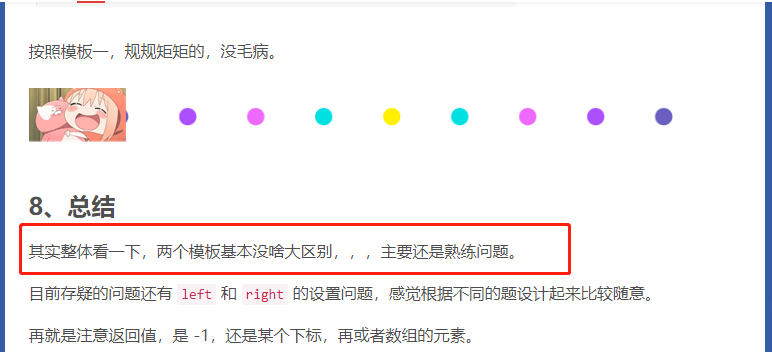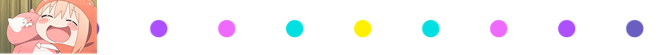## 2、题目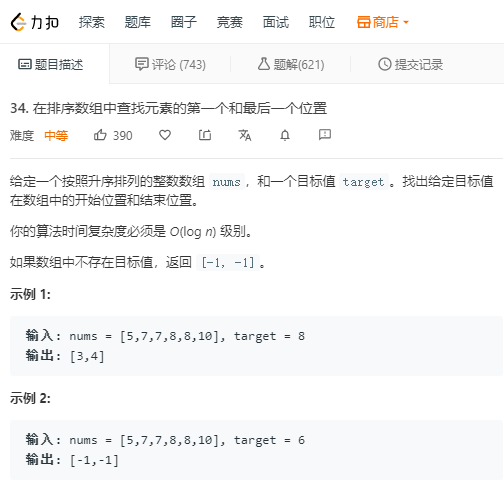## 3、正文

int left=0;
int right=nums.size()-1;
while(left<right){
int mid=left+right>>1;
if(nums[mid]>=target){
right=mid;
}
else{
left=mid+1;
}
}
return left;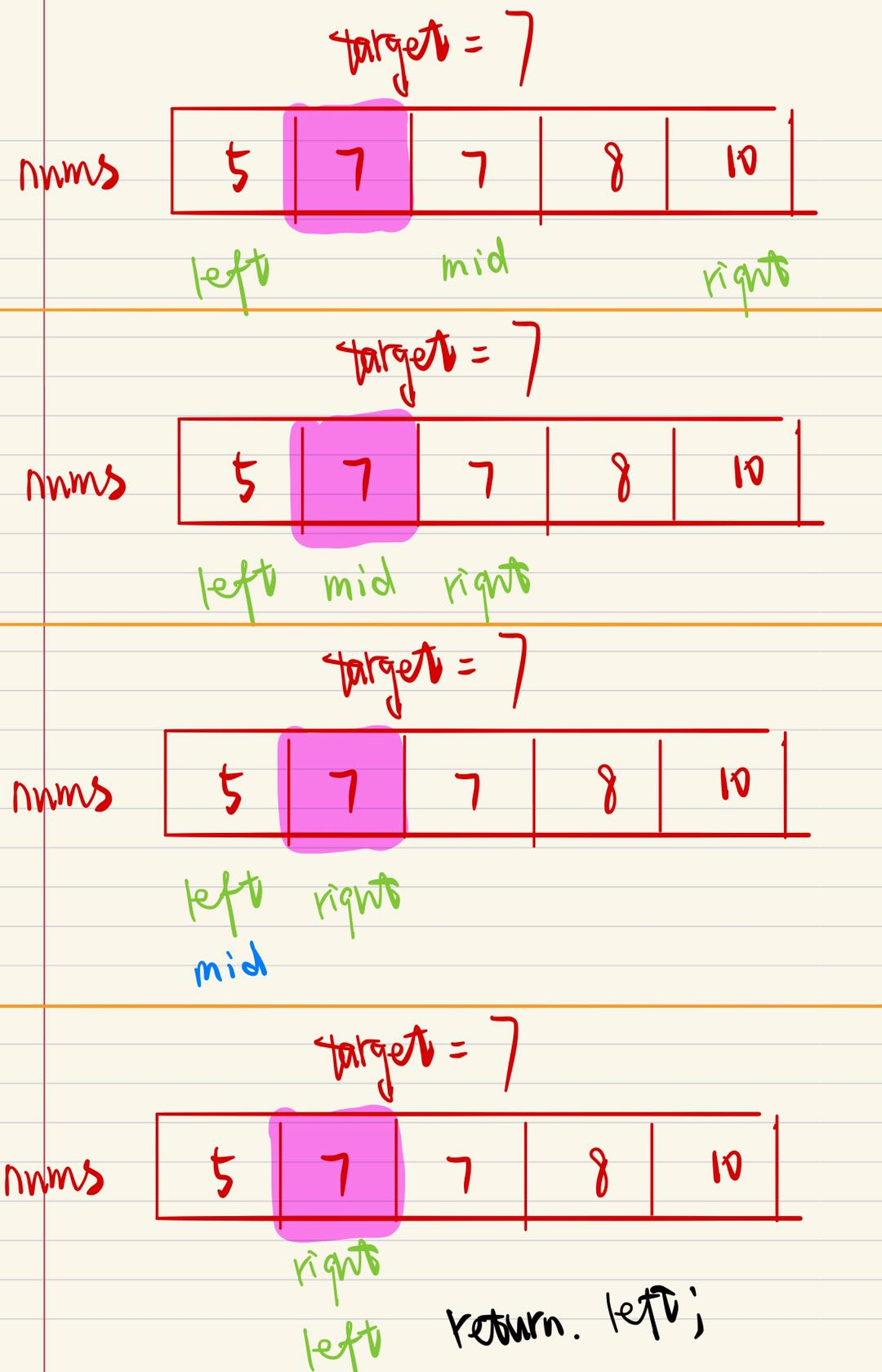int left=0;
int right=nums.size()-1;
while(left<right){
int mid=(left+right+1)>>1;
if(nums[mid]<=target){
left=mid;
}
else{
right=mid-1;
}
}
return right;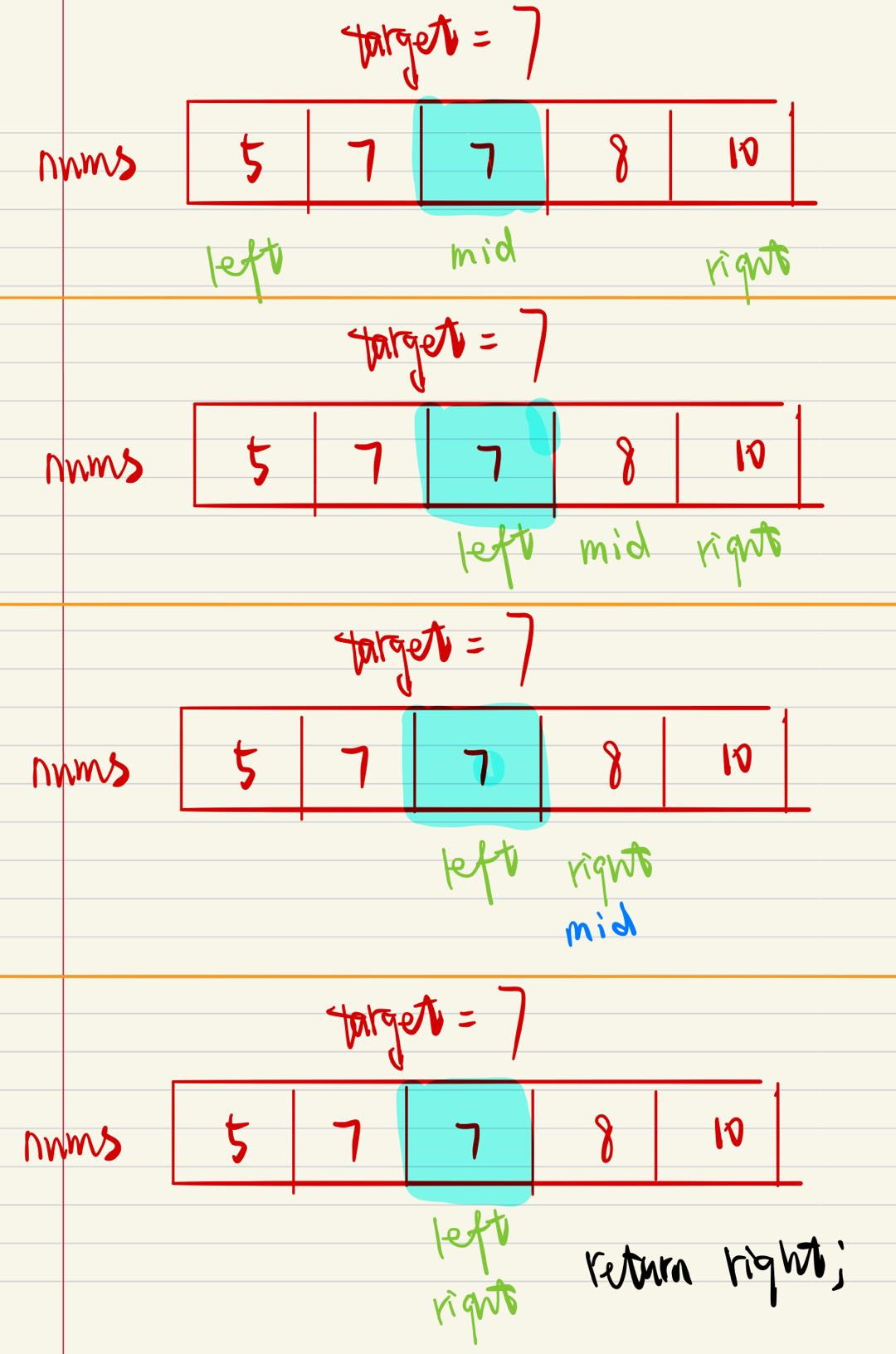## 4、代码

class Solution {
public:
vector<int> searchRange(vector<int>& nums, int target) {
if(nums.empty()) return {-1,-1};

int left=0;
int right=nums.size()-1;
while(left<right){
int mid=(left+right)>>1;
if(nums[mid]>=target){
right=mid;
}
else{
left=mid+1;
}
}
if(nums[left]!=target) return {-1,-1};
int start=left;

left=0;
right=nums.size()-1;
while(left<right){
int mid=(left+right+1)>>1;
if(nums[mid]<=target){
left=mid;
}
else{
right=mid-1;
}
}
if(nums[left]!=target) return {-1,-1};
int end=right;

return {start,end};
}
};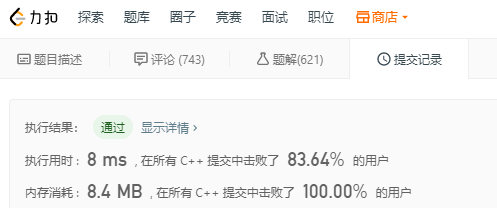04-22
04-27
03-1049万+
02-1950万+